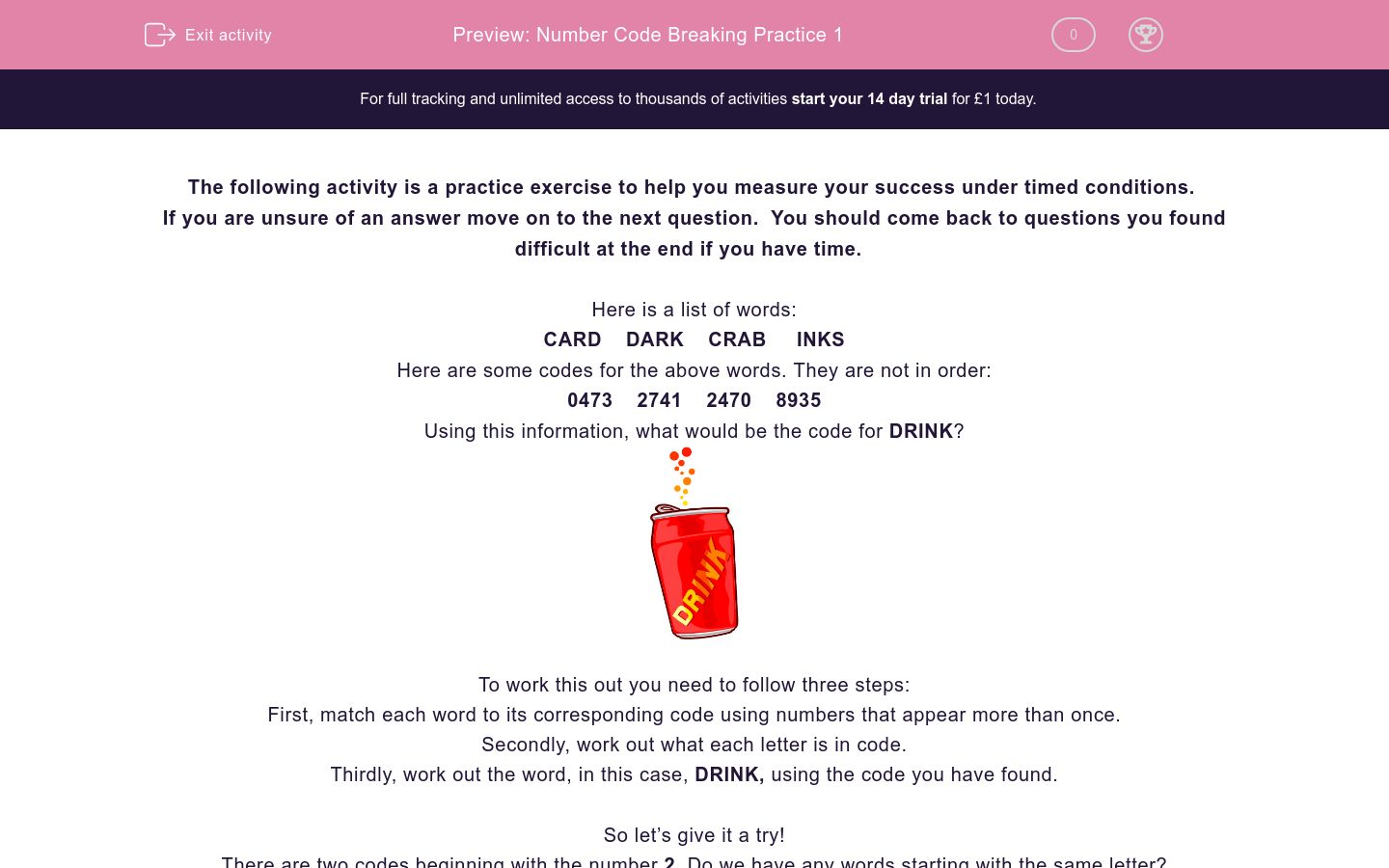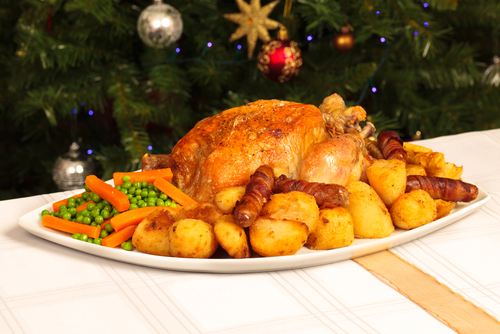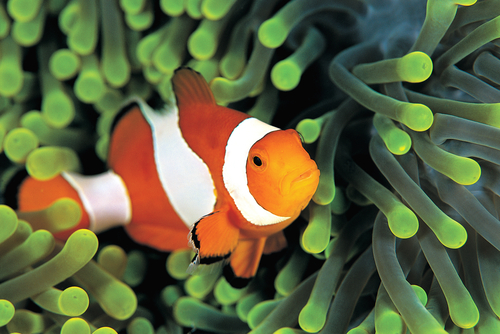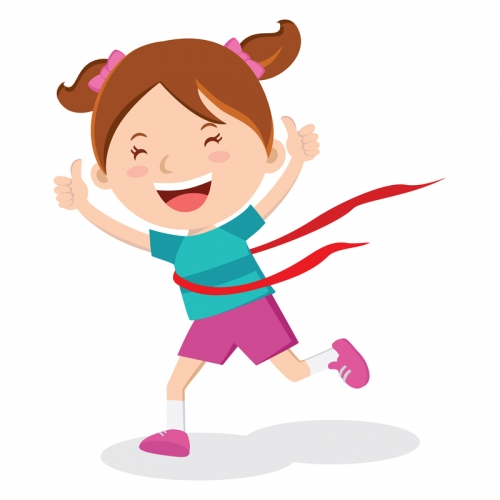# Number Code Breaking Practice 1

In this practice exercise, students will turn words into number codes using multiple words and codes to find and apply the pattern. It will develop their encoding and problem-solving skills under timed conditions.Key stage:  KS 2

Curriculum topic:   Exam-Style Questions: Verbal Reasoning

Curriculum subtopic:   Exam-Style Questions: Number Code Breaking

Difficulty level:### QUESTION 1 of 10

If you are unsure of an answer move on to the next question.  You should come back to questions you found difficult at the end if you have time.

Here is a list of words:

CARD    DARK    CRAB     INKS

Here are some codes for the above words. They are not in order:

0473    2741    2470    8935

Using this information, what would be the code for DRINK?To work this out you need to follow three steps:

First, match each word to its corresponding code using numbers that appear more than once.

Secondly, work out what each letter is in code.

Thirdly, work out the word, in this case, DRINK, using the code you have found.

So let’s give it a try!

There are two codes beginning with the number 2. Do we have any words starting with the same letter?

We have two words starting with the letter C, so 2 must represent C (2=C).

We have two codes with the number 4 in the second letter position. Which letter appears in the second letter position twice? 4 must be the letter A (4=A).

We now have enough information to match each word to a code, giving us:

DARK = 0473

CRAB = 2741

CARD = 2470

INKS = 8935

So D = 0, R = 7, I = 8, N = 9, K = 3.

If we use this code on the word DRINK, we get the answer of 07893.

Now it's time to begin this practice exercise.  Good Luck!

Welcome back to number code cracking, code cracker! This time I'll be challenging you to tackle some more advanced vocabulary - I know you can do it!

Here is a list of words:

TILL  DULL  TIDE  GATE

Here are some codes for the above words. They are not necessarily in order:

5733  2033  2054  9624

Using this information, what would be the code for ALTITUDE?63754202

63202754

75420263

63202457

Right then, code cracker, are you ready to have another go at this slightly trickier skill? I think you are!

Here is a list of words:

QUEUE  RENTS  SPIRE  QUEST

Here are some codes for the above words. They are not necessarily in order:

94105  72424  58394  72450

Using this information, what would be the code for REQUIRE?9743294

9472294

8472394

9472394

OK, code cracker, now take your time and look out for any similar letters!

Here is a list of words:

Here are some codes for the above words. They are not necessarily in order:

5923  5904  6913  4913

Using this information, what would be the code for SELDOM?635491

635094

653914

535491

We're on a roll, code cracker! Keep going - remember to go through this carefully and don't rush. You are still learning to master this skill, after all!

Here is a list of words:

INTER  SENDS  DENTS  DINESHere are some codes for the above words. They are not necessarily in order:

29410  61936  31946  32916

Using this information, what would be the code for INTENSE?

291961

2941619

2941961

9941991

Here, you're going to need to find the code for ABSURD, code cracker? Sometimes number code cracking can feel a bit of an absurd way to spend your time, but you'll be pleased you did when the exams come round!

Here is a list of words:

DUES  SURE  BARE  RUES

Here are some codes for the above words. They are not necessarily in order:

6912  2961  7061  5912

Using this information, what would be the code for ABSURD?072965

072966

072995

772955

Come on then, code cracker - onto another! Can you do it? Absolutely!

Here is a list of words:

MUSE  STEW  WHIM  EXITHere are some codes for the above words. They are not necessarily in order:

3760  1035  4913  5264

Using this information, what would be the code for SUMMIT?

196640

194460

199460

606449

Now you need to find the code for MISCHIEF, code cracker. I hope you don't plan on causing any mischief when your number code cracking adventure is done!

Here is a list of words:

CHOSE  FISH  MICE  WISEHere are some codes for the above words. They are not necessarily in order:

92134  7532  6594  0534

Using this information, what would be the code for MISCHIEF?

65254793

69332547

65392547

56392547

I've given you quite a long word to work out the code for here, code cracker. Take your time and stay calm - you have definitely got this!

Here is a list of words:

CASE  POST  ICON  SOME

Here are some codes for the above words. They are not necessarily in order:

0248  4256  3146  7329

Using this information, what would be the code for COMPASSION?3250141729

3250144729

3254729141

3254729114

Two questions to go - you can do it, code cracker!

Here is a list of words:

RACE  CODE  HOPE  TAPEHere are some codes for the above words. They are not necessarily in order:

9032  3462  1472  5072

Using this information, what would be the code for CHARACTER?

310903529

319003529

209035293

210903529

You did it, code cracker! Wahoo! Brilliant work. Now, let's work carefully through this last one and end on a high!Here is a list of words:

PILL  DULL  COAT  PEAT

Here are some codes for the above words. They are not necessarily in order:

3622  7058  9122  9458

Using this information, what would be the code for DUPLICATE?

396712584

369721485

369217584

639217548

• Question 1

Welcome back to number code cracking, code cracker! This time I'll be challenging you to tackle some more advanced vocabulary - I know you can do it!

Here is a list of words:

TILL  DULL  TIDE  GATE

Here are some codes for the above words. They are not necessarily in order:

5733  2033  2054  9624

Using this information, what would be the code for ALTITUDE?63202754
EDDIE SAYS
OK, code cracker. These questions can take a bit longer to explain. Let's go through it step by step. 1. Match each word to its corresponding code using numbers that appear more than once: There are two codes beginning with the number 2. We have two words starting with the letter T, so 2 must represent T (2 = T). We have two codes with the number 3 repeated twice at the end of the word. The letter L is repeated twice at the end of two words. So, 3 must be the letter L (3 = L). We now have enough information to match each word to a code, giving us: TILL = 2033 DULL = 5733 TIDE = 2054 GATE = 9624 2. Work out what each letter is in code: So A = 6, L = 3, T = 2, I = 0, T = 2, U = 7, D = 5, E = 4 3. Work out the word: If we use this code on the word ALTITUDE, we get the answer of 63202754. I hope that makes sense! Take your time over these questions - they can be tricky at first, but you'll get there!
• Question 2

Right then, code cracker, are you ready to have another go at this slightly trickier skill? I think you are!

Here is a list of words:

QUEUE  RENTS  SPIRE  QUEST

Here are some codes for the above words. They are not necessarily in order:

94105  72424  58394  72450

Using this information, what would be the code for REQUIRE?9472394
EDDIE SAYS
How did you get on with this one, code cracker? Make sure that you write out the words and codes to help you - this makes it so much easier! 1. Match each word to its corresponding code using numbers that appear more than once: There are two codes beginning with the number 7. We have two words starting with the letter Q, so 7 must represent T (7 = Q). We have two codes with the number 2 in the second letter position. The letter U appears in the second letter position twice. So 2 must be the letter U. We now have enough information to match each word to a code, giving us: QUEUE = 72424 RENTS = 94105 SPIRE = 58394 QUEST = 72450 2. Work out what each letter is in code: So R = 9, E = 4, Q = 7, U = 2, I = 3, R = 9, E = 4 3. Work out the word: If we use this code on the word REQUIRE, we get the answer of 9472394. Take your time to go through the explanation a few times if you need, code cracker - slow and steady wins the race!
• Question 3

OK, code cracker, now take your time and look out for any similar letters!

Here is a list of words:

Here are some codes for the above words. They are not necessarily in order:

5923  5904  6913  4913

Using this information, what would be the code for SELDOM?635491
EDDIE SAYS
Did you spot that the letter E appeared a few times, code cracker? This would be a brilliant starting point if so. Follow the usual technique to help you: 1. Match each word to its corresponding code using numbers that appear more than once: There are three codes ending with the number 3, so 3 must represent E (3 = E) We have two codes in which the number 1 appears in the position of the third letter. So 1 must represent M (1 = M) We have two words which begin with the letter L and two codes which begin with the number 5. So, 5 = L. We now have enough information to match each word to a code, giving us: DOME = 4913 LOAD = 5904 SOME = 6913 LONE = 5923 2. Work out what each letter is in code: So S = 6, E = 3, L = 5, D = 4, O = 9, M = 1 3. Work out the word: If we use this code on the word SELDOM, we get the answer of 635491. Are you ready for another go at cracking these codes, code cracker? Let's move on to the next question!
• Question 4

We're on a roll, code cracker! Keep going - remember to go through this carefully and don't rush. You are still learning to master this skill, after all!

Here is a list of words:

INTER  SENDS  DENTS  DINESHere are some codes for the above words. They are not necessarily in order:

29410  61936  31946  32916

Using this information, what would be the code for INTENSE?

2941961
EDDIE SAYS
I hope you didn't find that too INTENSE, code cracker! If you follow the method and work through each stage carefully, you can get there. Check the explanation to help you! 1. Match each word to its corresponding code using numbers that appear more than once: There are two codes beginning with the number 3 and we have two words starting with the letter D, so 3 must represent D (3 = D). We have three codes ending with 6 and three words which end with S, so 6 = S. The number 9 appears in the position of the third letter twice. So 9 = N. We now have enough information to match each word to a code, giving us: INTER = 29410 SENDS = 61936 DENTS = 31946 DINES = 32916 2. Work out what each letter is in code: So I = 2, N = 9, T = 4, E = 1, N = 9, S = 6, E = 1 3. Work out the word: If we use this code on the word INTENSE, we get the answer of 2941961.
• Question 5

Here, you're going to need to find the code for ABSURD, code cracker? Sometimes number code cracking can feel a bit of an absurd way to spend your time, but you'll be pleased you did when the exams come round!

Here is a list of words:

DUES  SURE  BARE  RUES

Here are some codes for the above words. They are not necessarily in order:

6912  2961  7061  5912

Using this information, what would be the code for ABSURD?072965
EDDIE SAYS
Follow the usual technique and go stage by stage code cracker: 1. Match each word to its corresponding code using numbers that appear more than once: There are two codes ending with the number 2. Therefore, 2 must represent the letter S (2 = S). We have two codes in which the number 1 appears in the position of the third letter. So 1 represents the letter E (1 = E). We have two codes with the number 6 in the position of the third letter. So the number 6 represents the letter R (6 = R). We now have enough information to match each word to a code, giving us: DUES = 5912 SURE = 2961 BARE = 7061 RUES = 6912 2. Work out what each letter is in code: So A = 0, B = 7, S = 2, U = 9, R = 6, D = 5 3. Work out the word: If we use this code on the word ABSURD, we get the answer of 072965. Can you see how we got there? Read through the explanation a couple of times if you need to before you move on - this will help you feel more confident with the next question!
• Question 6

Come on then, code cracker - onto another! Can you do it? Absolutely!

Here is a list of words:

MUSE  STEW  WHIM  EXITHere are some codes for the above words. They are not necessarily in order:

3760  1035  4913  5264

Using this information, what would be the code for SUMMIT?

194460
EDDIE SAYS
Did you spot that the letter E was repeated in a few words and that the number 3 was repeated in a few codes, code cracker? This will have given you a great starting point if so! 1. Match each word to its corresponding code using numbers that appear more than once: The number 3 appears three times in various positions (fourth letter, third letter, first letter). So number 3 must represent the letter E (3 = E). The number 4 appears once in the position of the first letter and once in the position of the last letter. Therefore, the number 4 must represent the letter M. We now have enough information to match each word to a code, giving us: MUSE = 4913 STEW = 1035 WHIM = 5264 EXIT = 3760 2. Work out what each letter is in code: So S = 1, U = 9, M = 4, M = 4, I = 6, T = 0 3. Work out the word: If we use this code on the word SUMMIT, we get the answer of 194460.
• Question 7

Now you need to find the code for MISCHIEF, code cracker. I hope you don't plan on causing any mischief when your number code cracking adventure is done!

Here is a list of words:

CHOSE  FISH  MICE  WISEHere are some codes for the above words. They are not necessarily in order:

92134  7532  6594  0534

Using this information, what would be the code for MISCHIEF?

65392547
EDDIE SAYS
Did you work out the code for MISCHIEF, code cracker? If not, don't be afraid to have another go - practice ensures progress! 1. Match each word to its corresponding code using numbers that appear more than once: Did you spot that one of the words is made up of five letters and the others are all four letters? This makes it easy to spot which one is the code for CHOSE - it has to be 92134. Having worked that out, it is easy to sort out all the others because we now know the code for c, h, o, s and e! We now have enough information to match each word to a code, giving us: CHOSE = 92134 FISH = 7532 MICE = 6594 WISE = 0534 2. Work out what each letter is in code: So M = 6, I = 5, S = 3, C = 9, H = 2, I = 5, E = 4, F = 7 3. Work out the word: If we use this code on the word MISCHIEF, we get the answer of 65392547. Great stuff, code cracker - let's move on to another question!
• Question 8

I've given you quite a long word to work out the code for here, code cracker. Take your time and stay calm - you have definitely got this!

Here is a list of words:

CASE  POST  ICON  SOME

Here are some codes for the above words. They are not necessarily in order:

0248  4256  3146  7329

Using this information, what would be the code for COMPASSION?3250144729
EDDIE SAYS
If you didn't get it right, code cracker, don't worry. Show yourself a bit of COMPASSION! We all have to start somewhere. Check through the explanation carefully. I'm here to help! 1. Match each word to its corresponding code using numbers that appear more than once: There are two codes ending with the number 6 . We have two words ending with the letter E, so 6 must represent E (6 = E). We have two codes with the number 4 in the third letter position. The letter S appears in the third letter position twice. So 4 must be the letter S (4 = S). We now have enough information to match each word to a code, giving us: CASE = 3146 POST = 0248 ICON = 7329 SOME = 4256 2. Work out what each letter is in code: So C = 3, O = 2, M = 5, P = 0, A = 1, S = 4, S = 4, I = 7 , O = 2, N = 9 3. Work out the word: If we use this code on the word COMPASSION, we get the answer of 3250144729.
• Question 9

Two questions to go - you can do it, code cracker!

Here is a list of words:

RACE  CODE  HOPE  TAPEHere are some codes for the above words. They are not necessarily in order:

9032  3462  1472  5072

Using this information, what would be the code for CHARACTER?

310903529
EDDIE SAYS
Did you show good CHARACTER and persevere through this question, code cracker? Keep that effort up right until the end! 1. Match each word to its corresponding code using numbers that appear more than once: We have four codes ending with the number 2. All the words end with the letter E. So 2 must be E (2 = E). We have two codes with the number 4 in the second letter position. The letter O appears in the second letter position twice. So 4 must be the letter O (4 = O). We have the number 3 appearing in two codes, as the first letter in one code and in the third letter position in the other. The letter C appears as the first and the third letters so 3 must be C. (3 = C). We now have enough information to match each word to a code, giving us: RACE = 9032 CODE = 3462 HOPE = 1472 TAPE = 5072 2. Work out what each letter is in code: So C = 3, H = 1, A = 0, R = 9, A = 0, C = 3, T = 5, E = 2, R = 9 3. Work out the word: If we use this code on the word CHARACTER, we get the answer of 310903529. I hope that clears up any confusion - if you check your work alongside the explanation, you should be able to notice where you made any mistakes.
• Question 10

You did it, code cracker! Wahoo! Brilliant work. Now, let's work carefully through this last one and end on a high!Here is a list of words:

PILL  DULL  COAT  PEAT

Here are some codes for the above words. They are not necessarily in order:

3622  7058  9122  9458

Using this information, what would be the code for DUPLICATE?

369217584
EDDIE SAYS
How did you get on with that last question, code cracker? Will you bear with me whilst we go through one final explanation? It will be worth it in the end! 1. Match each word to its corresponding code using numbers that appear more than once: There are two codes ending with the letter 2 used twice in a row (22). There are two words which end with a double letter (LL). So 2 must be L (2 = L). We have two words beginning with the number 9 and two words which begin with the letter P. Therefore, 9 must be P (9 = P). We now have enough information to match each word to a code, giving us: PILL = 9122 DULL = 3622 COAT = 7058 PEAT = 9458 2. Work out what each letter is in code: So D = 3, U = 6, P = 9, L = 2, I = 1, C = 7, A = 5, T = 8, E = 4 3. Work out the word: If we use this code on the word DUPLICATE, we get the answer of 369217584. And you're done! High five!
---- OR ----

Sign up for a £1 trial so you can track and measure your child's progress on this activity.

### What is EdPlace?

We're your National Curriculum aligned online education content provider helping each child succeed in English, maths and science from year 1 to GCSE. With an EdPlace account you’ll be able to track and measure progress, helping each child achieve their best. We build confidence and attainment by personalising each child’s learning at a level that suits them.

Get started